## conference papersJOURNAL OFAPPLIEDCRYSTALLOGRAPHY
ISSN: 1600-5767
Volume 40| Part s1| April 2007| Pages s175-s178

## Collapse of the hydration shell of a protein prior to thermal unfolding

aDepartment of Physics, Gunma University, Maebashi 371-8510, Japan, and bJapan Synchrotron Radiation Research Institute, Sayo Hyogo 679-5198, Japan
*Correspondence e-mail: mhirai@fs.aramaki.gunma-u.ac.jp

(Received 16 August 2006; accepted 22 January 2007; online 21 April 2007)

Based on high statistical quality wide-angle X-ray scattering data for the unfolding–refolding process of hen egg-white lysozyme (HEWL), we have analysed the change of the hydration shell as a function temperature using the program CRYSOL. The present results suggest that the decrease of the hydration-shell density starts from a lower temperature than the transition temperature of the collapse of the tertiary structure of HEWL. Although the use of CRYSOL for scattering data for proteins before the transition has an apparent limitation, the collapse of the hydration shell prior to the unfolding of HEWL agrees with a slight tendency of the radius of gyration to decrease during the thermal unfolding process.

### 1. Introduction

Hydration of biological macromolecules is assumed to have an important role in their structural stability and functions (Israelachvili & Wennerström, 1996). As suggested by inelastic neutron scattering experiments, the functional properties and dynamics of proteins in solution are coupled with the dynamic behaviour of the surrounding water molecules (Gabel et al., 2002). Studies of proteins using X-ray and neutron solution scattering techniques indicate that the density of water around the protein surface is much higher than that of bulk water (Svergun et al., 1998). They showed the existence of a hydration shell whose average density is around 10% larger than that of the bulk water. Molecular dynamics simulation studies also suggested that the variation in the first hydration shell density is subject to the electrostatic properties of the protein surface and local surface topography (Makarov et al., 1998, 2000; Dastidar & Mukhopadhyay, 2003). However, the properties of the hydrated water shells are still the subject of many experimental and theoretical investigations.

On the other hand, the mechanism by which proteins fold into their native structures is one of essential problems in biology and is still under extensive study (Pain, 2000; Oliveberg & Wolynes, 2006). To clarify the mechanism of protein folding, various methods have been used to observe detailed features of the folding–unfolding processes of proteins (Dobson, 2004). Hydration of proteins is assumed to greatly contribute not only to their structural stability but also their functions. By using synchrotron radiation (SR) wide-angle X-ray scattering and differential scanning calorimetry (DSC), we have been studying the thermal unfolding–refolding process of hen egg-white lysozyme (HEWL) at various pH values under stepwise heating and cooling conditions (Hirai et al., 1998, 1999; Arai & Hirai, 1999). Recently we demonstrated that the wide-angle X-ray scattering (WAXS) method using high-intensity X-rays from a third-generation synchrotron-radiation source enables us to observe directly the whole hierarchical structure of proteins including quaternary, tertiary, domain and secondary structures in solution (Hirai et al., 2002), and we showed that the structures of proteins in solutions can be compared with crystallographic structures by using a program such as CRYSOL (Svergun et al., 1995). By using SR-WAXS we successfully analysed the details of the reversible unfolding–refolding process of HEWL covering all the hierarchical structures from tertiary to secondary structure (over the real-space distance from ~2.5 to ~125 Å), and showed that the pH dependence of the thermal structural transition of HEWL is characterized by its hierarchical structures and the cooperativity between them (Hirai et al., 2004). In that report we presented a new hierarchical map of unfolding–refolding transitions by determining the molar ratios of the native-structure protein for each hierarchical level at an intermediate transition state, which would be comparable to the fraction of native contacts used in a `folding funnel' representation (Dill & Chan, 1997; Dinner et al., 2000). Thus, the SR-WAXS method is widely applicable to the studies of various proteins (Fischetti et al., 2003, 2004) and enables discussion of the relation between the structural transitions and intramolecular structure of proteins (Koizumi et al., 2006).

In the present report we have evaluated the change of the hydration shell during the thermal unfolding–refolding transition by using the CRYSOL program to analyse the WAXS data of HEWL at the transition. We have found that the initial stage of HEWL unfolding accompanies a collapse of the hydration shell. In the present analyses we have used the WAXS data reported previously (Hirai et al., 2004). We will also discuss the feasibility and limitation of the present analysis.

### 2. Materials and method

#### 2.1. Sample preparation

Three-times crystallized HEWL from Sigma Chemical Co. was used for WAXS measurements. HEWL was dissolved in water whose pH was adjusted by adding HCl before the dissolution. In many cases of protein-folding studies, an aggregation of proteins occurs in the unfolding and/or refolding process. As reported previously (Hirai et al., 1998, 1999), the present solvent conditions can avoid such aggregation due to the repulsive interparticle interaction between proteins through the Coulomb potential. Final pH values of the protein solutions were determined by using a digital HM-60V pH meter from TOA Electronics Ltd. The concentrations of the samples were 0.05 g ml−1 for the thermal unfolding–refolding measurements and 0.01 g ml−1 for the pH dependence measurements.

#### 2.2. Small-angle and wide-angle X-ray scattering measurements

The spectrometer used for SR-WAXS experiments was an X-ray scattering spectrometer installed at BL-40B2 of the 8 GeV synchrotron-radiation source of the Japan Synchrotron Radiation Research Institute (JASRI), Harima, Japan. Details of the spectrometer have been described elsewhere (Miura et al., 2000). The sample-to-detector distance and the X-ray wavelength used were 41 cm and 0.729 Å, respectively. The scattering intensity was recorded by an R-AXIS IV imaging plate system from Rigaku. The intensities of the incident and transmitted X-rays were monitored by a pair of ionization chambers. A sample cell composed of a pair of thin quartz windows with 1 mm path length was used. The exposure time to the X-rays was 30 s for each measurement. The q values of the circularly averaged scattering intensity I(q) were calibrated using the diffraction peaks of silver behenate (Huang et al., 1993), where q = (4π/λ)sin θ, λ is the wavelength and 2θ is the scattering angle. The beam size at the sample position was ~0.1 mm2. Other details of the WAXS measurements are given in the previous reports (Hirai et al., 2002, 2004).

#### 2.3. Scattering data treatment and model analysis

To obtain the net scattering from the proteins the following equation was used (Hirai et al., 2002, 2004).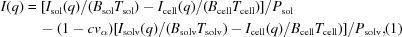where Isol(q), Isolv(q), Icell(q), Bsol, Bsolv, Bcell, Tsol, Tsolv and Tcell are the observed scattering intensities, the incident beam intensities and the transmissions of the solution and the solvent at each temperature and the sample cell, respectively. Psol and Psolv are the water-correlation peak intensities of the solution and the solvent; c and vα are the concentration of the protein molecule and its partial specific volume, respectively. The vα value used was 0.712 ml g−1 for HEWL (Gekko & Hasegawa, 1986). In the present modelling analysis we used the CRYSOL program. This program is well known for fitting experimental scattering curves of proteins in solutions based on their crystallographic coordinates by considering the hydration shell (Svergun et al., 1995, 1998). WAXS data in the q range from 0.09 to 1.8 Å−1 were used for fitting based on the crystallographic data of HEWL [6LYZ from Diamond (1974)] using the CRYSOL program using 50 spherical harmonics and default parameters. Therefore, the only fitting parameter was the hydration shell density (HSD).

### 3. Results and discussion

#### 3.1. Concentration and pH dependence of the hydration shell density

As we used relatively high concentration protein solutions for obtaining high statistical quality WAXS data, we have checked the effect of the protein concentration on the HSD value by using CRYSOL with previous data for HEWL (Hirai et al., 1998). Fig. 1A shows the concentration dependence of the HSD and χ values obtained, and Fig. 1B shows the scattering data from 0.01 to 0.2 g ml−1 HEWL in 50 mM Hepes at pH 7 at 293 K (Hirai et al., 1998). The χ value is defined by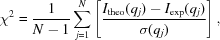where N is the number of experimental data points, Itheo(q) and Iexp(q) are the theoretical and experimental intensities, and σ(q) is the standard deviation of Iexp(q) (Svergun et al., 1998).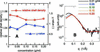Figure 1 (A) Concentration dependence of the HSD and χ values. (B) Scattering data from the HEWL solutions used for HSD estimation, with concentrations ranging from 0.01 to 0.2 g ml−1 in 50 mM Hepes at pH 7 at 293 K (reproduced from Hirai et al., 1998).

In Fig. 1A the estimated HSD values below a concentration of 0.05 g ml−1 are comparable to the reported value of 1.07 (Svergun et al., 1998) in spite of the use of high-q data (q > 0.09 Å−1), where the average scattering density of water was taken as 1.0. Fig. 2A shows the pH dependence of the HSD and radius of gyration Rg of 0.01 g ml−1 HEWL, and Fig. 2B shows the WAXS data used for the calculation (Hirai et al., 2004). As shown in Fig. 2A, the values of the HSD and Rg are mostly independent of pH in the wide pH range from 1.9 to 6.3, and give reasonable values of ~1.07 and 15.4 ± 0.2 Å, respectively. Figs. 1A and 2A suggest the scattering data above q = 0.09 Å−1 reflect the contribution of the hydration shell well and are enough for estimating the hydration shell density and Rg using CRYSOL in the case of HEWL. This situation can be understood from Fig. 3. Fig. 3is the theoretical WAXS curve and Rg value of HEWL depending on the relative value of the average excess scattering density, the so-called contrast of the hydration shell defined by (HSD − 1.0), which was obtained by CRYSOL. The increase of the HSD from 1.0 to 1.06 increases Rg from 14.01 to 15.30 Å and changes the scattering profile below ~0.8 Å−1. The experimental Rg value of 15.4 ± 0.2 Å at 298 K at pH 4.6 corresponds to the theoretical Rg value at the relative shell contrast of 0.068, which is in agreement with the reported value (Svergun et al., 1998). Thus, WAXS curves above q = 0.09 Å−1 are assumed to reflect the change of the HSD well in spite of the use of relatively high q values. It should be mentioned that the change of the shell contrast does not affect the theoretical WAXS curves above q = ~1 Å−1.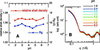Figure 2 (A) pH dependence of the HSD and radius of gyration Rg of 0.01 g ml−1 solutions of HEWL at 298 K. (B) WAXS data used for the calculations (reproduced from Hirai et al., 2004).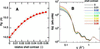Figure 3 (A) Radius of gyration of HEWL as a function of the contrast of the hydration shell. (B) Shell-contrast dependence of the theoretical WAXS curves. (A) and (B) were obtained from CRYSOL using data for 6LYZ. The contrast was varied from 0 to 0.06.

On the other hand, in the case of the presence of interparticle interaction between solute particles the Rg value evaluated using a Guinier plot [lnI(q) versus q2] is known to be sensitive to the q range used for calculation. Fig. 4shows the concentration dependence of Rg values estimated by a Guinier plot using different q ranges. Fig. 4suggests that the effect of repulsive interparticle interaction on the scattering curve appearing as a broad peak below q = ~0.065 Å−1 for 0.05 g ml−1 can be neglected when we use the scattering data above q = 0.09 Å−1, which also confirms that the use of a relatively high q value is reasonable to avoid the effect of interparticle interaction on scattering data analysis for high concentration samples.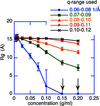Figure 4 Radius of gyration, Rg, of HEWL (pH 7.0, 298 K) as a function of concentration. The Rg values were estimated by applying a Guinier plot to the scattering data for the different q ranges shown in the figure. The arrows indicate the Rg values that are indeterminable due to the presence of a broad interparticle correlation peak. The data used are from Arai & Hirai (1999).

#### 3.2. Temperature dependence of experimental WAXS curves compared with fitted theoretical curves

Fig. 5shows a part of the experimental WAXS curves of 0.05 g ml−1 HEWL on heating from 285 to 352 K (Hirai et al., 2004) compared with the fitted theoretical curves obtained using CRYSOL, where A, B and C correspond to pH 2.2, 3.6 and 4.5, respectively. In the temperature range 285–318 K the experimental and theoretical WAXS curves at pH 3.6 and 4.5 show good agreement over the whole q range. On the other hand, the theoretical WAXS curve at pH 2.2 shows a slight deviation from the experimental one even at 285 K. At all pH values the deviation becomes evident above the transition temperature as shown in §3.3. Thus, Fig. 5indicates that the fitting procedure using CRYSOL would be applicable to the WAXS data especially at pH 3.6 and 4.5 below ~318 K, suggesting that the relative value of the HSD estimated by CRYSOL would be reasonable below this temperature.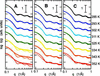Figure 5 Comparison of the experimental WAXS curves of 0.05 g ml−1 HEWL at different temperatures with the fitted theoretical WAXS curves obtained from CRYSOL. (A) at pH 2.2; (B) at pH 3.6; (C) at pH 4.5. The dots and thick lines correspond to the experimental data and the fitted theoretical WAXS curves, respectively. The experimental data are reproduced from Hirai et al. (2004).

#### 3.3. Temperature dependence of χ and the hydration shell density of HEWL at unfolding and refolding

Based on the above consideration, we have evaluated the change of the relative value of the HSD of HEWL in the thermal unfolding and refolding processes by using CRYSOL with all WAXS data reported previously (Hirai et al., 2004). Figs. 6A and 6B show the temperature dependence of the relative value of the HSD at different pH values and the χ value obtained by CRYSOL, where A and B correspond to the heating (unfolding) and cooling (refolding) processes, respectively. As reported previously, the onset (Ton) and midpoint (Tm) temperatures of the HEWL thermal transition observed by DSC agree well with the structural transition temperatures observed from the change of Rg (Hirai et al., 1999), and the thermal unfolding and refolding processes of HEWL are highly reversible (Arai & Hirai, 1999; Hirai et al., 2004). The Ton and Tm of Rg observed were Ton = ~339 K and Tm = ~349 K for pH 4.5, Ton = ~335 K and Tm = ~346 K for pH 3.6, Ton = ~332 K and Tm = ~344 K for pH 3.1, and Ton = ~318 K and Tm = ~326 K for pH 2.2 (Hirai et al., 2004). As shown in Figs. 6A and 6B, the change of the HSD is mostly reversible both in the thermal unfolding and refolding processes. The significant increase of the χ value starts from around Ton at every pH value, especially at pH 4.5 and 3.6. It is clear that the relative values of the HSD above the temperature at which the χ value shows a significant increase are meaningless. On the other hand, even below Ton, where the change of the χ value is relatively small, the relative value of the HSD estimated shows a decreasing tendency for all pH values. This tendency agrees with the finding of a slight decrease of Rg below Ton reported previously (Hirai et al., 1998, 1999). Thus, Fig. 6suggests that before the main transition of the tertiary structure of HEWL a collapse or change of the hydration shell begins. Alternatively, the elevation of temperature affects at first the change of the hydration shell through the enhancement of the thermal fluctuation of the protein, destabilizes the native folded structure, and finally induces the collapse of the tertiary structure with an accompanying significant increase of Rg and a large change of Gibbs free energy (Hirai et al., 1998, 1999; Arai & Hirai, 1999).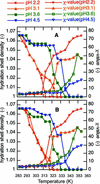Figure 6 Temperature dependence of the relative value of the hydration shell density and the χ value obtained by CRYSOL, where (A) and (B) correspond to the heating (unfolding) and cooling (refolding) processes, respectively.

The present interpretation would be reasonable as follows. The hydration shell of a protein results from different types of hydration, namely, electrostatic hydration by dissociation amino acids, hydrophobic hydration by non-polar amino acids, and hydration by hydrogen bonds by polar amino acids. Even below Ton, the thermal expansion of the tertiary structure due to the enhancement in the intramolecular fluctuation accompanies further exposure of the hydrophobic core within the protein, which would increase the hydrophobic hydration that decreases the HSD. Thus, the thermal expansion and the collapse of the hydrophilic hydration shell would contribute to the change of Rg in opposite ways. Alternatively, in the case of HEWL the thermal expansion and the collapse of the hydrophilic hydration shell would begin simultaneously; however, the latter effect on Rg would be dominant below Ton as found previously in the slight decrease of Rg (Hirai et al., 1998, 1999). The change of the HSD suggested in the present study might be confirmed by using other experimental and theoretical methods such as nuclear magnetic resonance and molecular dynamics simulation.

The present results suggest that the use of the program CRYSOL would be applicable not only to the comparison of protein structures in solutions with those in crystal states but also to the estimation of the changes of HSDs for small proteins for which the tertiary structures are mostly native-like. We should mention that the present analysis is not applicable to the case of the thermal unfolding of α-lactalbumin (α-LA), a homologous protein of HEWL, since the observed WAXS profile of α-LA in solution at room temperature is evidently different from the theoretical one in the crystal state calculated by CRYSOL (Koizumi et al., 2006). Thus, the reasonability of estimated HSDs must be judged both by the χ value obtained and by the agreement of the fitted theoretical WAXS curves with the experimental ones.

### Acknowledgements

The WAXS experiments were done under the approval of the JASRI Program Advisory Committee (Proposal Nos. 2001B0455 and 2004B0082).

### ReferencesJOURNAL OFAPPLIEDCRYSTALLOGRAPHY